# OpenCV 实现图像的腐蚀与膨胀

## 目标

• 如何使用OpenCV提供的两种最基本的形态学操作,腐蚀与膨胀( Erosion 与 Dilation):

## 原理

### 形态学操作

• 简单来讲，形态学操作就是基于形状的一系列图像处理操作。通过将 结构元素 作用于输入图像来产生输出图像。

• 最基本的形态学操作有二：腐蚀与膨胀(Erosion 与 Dilation)。 他们的运用广泛:

• 消除噪声
• 分割(isolate)独立的图像元素，以及连接(join)相邻的元素。
• 寻找图像中的明显的极大值区域或极小值区域。
• 通过以下图像，我们简要来讨论一下膨胀与腐蚀操作(译者注：注意这张图像中的字母为黑色，背景为白色，而不是一般意义的背景为黑色，前景为白色）: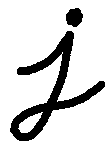卓小混混 3年前

#### 膨胀

• 此操作将图像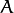与任意形状的内核 (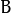)，通常为正方形或圆形,进行卷积。

• 内核有一个可定义的 锚点, 通常定义为内核中心点。

• 进行膨胀操作时，将内核划过图像,将内核覆盖区域的最大相素值提取，并代替锚点位置的相素。显然，这一最大化操作将会导致图像中的亮区开始”扩展” (因此有了术语膨胀 dilation )。对上图采用膨胀操作我们得到: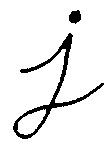卓小混混 3年前

#### 腐蚀

• 腐蚀在形态学操作家族里是膨胀操作的孪生姐妹。它提取的是内核覆盖下的相素最小值。

• 进行腐蚀操作时，将内核划过图像,将内核覆盖区域的最小相素值提取，并代替锚点位置的相素。

• 以与膨胀相同的图像作为样本,我们使用腐蚀操作。从下面的结果图我们看到亮区(背景)变细，而黑色区域(字母)则变大了。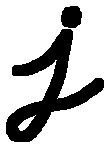卓小混混 3年前

## 源码

``````#include "opencv2/imgproc/imgproc.hpp"
#include "opencv2/highgui/highgui.hpp"
#include "highgui.h"
#include <stdlib.h>
#include <stdio.h>

using namespace cv;

/// 全局变量
Mat src, erosion_dst, dilation_dst;

int erosion_elem = 0;
int erosion_size = 0;
int dilation_elem = 0;
int dilation_size = 0;
int const max_elem = 2;
int const max_kernel_size = 21;

/** Function Headers */
void Erosion( int, void* );
void Dilation( int, void* );

/** @function main */
int main( int argc, char** argv )
{
src = imread( argv );

if( !src.data )
{ return -1; }

/// 创建显示窗口
namedWindow( "Erosion Demo", CV_WINDOW_AUTOSIZE );
namedWindow( "Dilation Demo", CV_WINDOW_AUTOSIZE );
cvMoveWindow( "Dilation Demo", src.cols, 0 );

/// 创建腐蚀 Trackbar
createTrackbar( "Element:\n 0: Rect \n 1: Cross \n 2: Ellipse", "Erosion Demo",
&erosion_elem, max_elem,
Erosion );

createTrackbar( "Kernel size:\n 2n +1", "Erosion Demo",
&erosion_size, max_kernel_size,
Erosion );

/// 创建膨胀 Trackbar
createTrackbar( "Element:\n 0: Rect \n 1: Cross \n 2: Ellipse", "Dilation Demo",
&dilation_elem, max_elem,
Dilation );

createTrackbar( "Kernel size:\n 2n +1", "Dilation Demo",
&dilation_size, max_kernel_size,
Dilation );

/// Default start
Erosion( 0, 0 );
Dilation( 0, 0 );

waitKey(0);
return 0;
}

/**  @function Erosion  */
void Erosion( int, void* )
{
int erosion_type;
if( erosion_elem == 0 ){ erosion_type = MORPH_RECT; }
else if( erosion_elem == 1 ){ erosion_type = MORPH_CROSS; }
else if( erosion_elem == 2) { erosion_type = MORPH_ELLIPSE; }

Mat element = getStructuringElement( erosion_type,
Size( 2*erosion_size + 1, 2*erosion_size+1 ),
Point( erosion_size, erosion_size ) );

/// 腐蚀操作
erode( src, erosion_dst, element );
imshow( "Erosion Demo", erosion_dst );
}

/** @function Dilation */
void Dilation( int, void* )
{
int dilation_type;
if( dilation_elem == 0 ){ dilation_type = MORPH_RECT; }
else if( dilation_elem == 1 ){ dilation_type = MORPH_CROSS; }
else if( dilation_elem == 2) { dilation_type = MORPH_ELLIPSE; }

Mat element = getStructuringElement( dilation_type,
Size( 2*dilation_size + 1, 2*dilation_size+1 ),
Point( dilation_size, dilation_size ) );
///膨胀操作
dilate( src, dilation_dst, element );
imshow( "Dilation Demo", dilation_dst );
}``````卓小混混 3年前

## 解释

1. 大部分代码应该不需要解释了 (如果有任何疑问，请回头参考前面的教程)。 让我们来回顾一下本程序的总体流程:

• 装载图像 (可以是 RGB图像或者灰度图 )
• 创建两个显示窗口 (一个用于膨胀输出,一个用于腐蚀输出)
• 为每个操作创建两个 Trackbars:
• 第一个 trackbar “Element” 返回 erosion_elem 或者 dilation_elem
• 第二个 trackbar “Kernel size” 返回 erosion_size 或者 dilation_size 。
• 每次移动标尺, 用户函数 Erosion 或者 Dilation 就会被调用，函数将根据当前的trackbar位置更新输出图像。

让我们分析一下这两个函数:

2. Erosion:

``````/**  @function Erosion  */
void Erosion( int, void* )
{
int erosion_type;
if( erosion_elem == 0 ){ erosion_type = MORPH_RECT; }
else if( erosion_elem == 1 ){ erosion_type = MORPH_CROSS; }
else if( erosion_elem == 2) { erosion_type = MORPH_ELLIPSE; }

Mat element = getStructuringElement( erosion_type,
Size( 2*erosion_size + 1, 2*erosion_size+1 ),
Point( erosion_size, erosion_size ) );
/// 腐蚀操作
erode( src, erosion_dst, element );
imshow( "Erosion Demo", erosion_dst );
}``````
• 进行 腐蚀 操作的函数是 erode 。 它接受了三个参数:

• src: 原图像

• erosion_dst: 输出图像

• element: 腐蚀操作的内核。 如果不指定，默认为一个简单的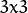矩阵。否则，我们就要明确指定它的形状，可以使用函数getStructuringElement:

``````Mat element = getStructuringElement( erosion_type,
Size( 2*erosion_size + 1, 2*erosion_size+1 ),
Point( erosion_size, erosion_size ) );``````

我们可以为我们的内核选择三种形状之一:

• 矩形: MORPH_RECT
• 交叉形: MORPH_CROSS
• 椭圆形: MORPH_ELLIPSE

然后，我们还需要指定内核大小，以及 锚点 位置。不指定锚点位置，则默认锚点在内核中心位置。

• 就这些了，我们现在可以对图像进行腐蚀操作了。

注意：

OpenCV的 erode 函数还有另外的参数，其中一个参数允许你一下对图像进行多次腐蚀操作。在这个简单的文档中没有用到它，但是你可以参考OpenCV的使用手册。

3. Dilation:卓小混混 3年前

``````/** @function Dilation */
void Dilation( int, void* )
{
int dilation_type;
if( dilation_elem == 0 ){ dilation_type = MORPH_RECT; }
else if( dilation_elem == 1 ){ dilation_type = MORPH_CROSS; }
else if( dilation_elem == 2) { dilation_type = MORPH_ELLIPSE; }

Mat element = getStructuringElement( dilation_type,
Size( 2*dilation_size + 1, 2*dilation_size+1 ),
Point( dilation_size, dilation_size ) );
/// Apply the dilation operation
dilate( src, dilation_dst, element );
imshow( "Dilation Demo", dilation_dst );
}``````卓小混混 3年前

## 结果

• 编译并使用图像路径作为参数运行程序，比如我们使用以下图像:下面是操作的结果。 更改Trackbars的位置就会产生不一样的输出图像，自己试试吧。 最后，你还可以通过增加第三个Trackbar来控制膨胀或腐蚀的次数。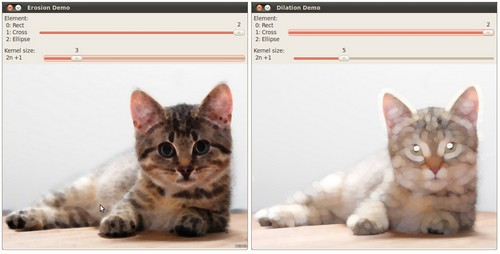卓小混混 3年前